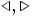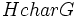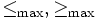# Notation for subgroup properties

•$\le, \ge$ denotes the tautology subgroup property, viz$H \le G$ or$G \ge H$ means that$H$ is a subgroup of$G$ (with no further restrictions)
•$\triangleleft, \triangleright$ denotes the subgroup property of being a normal subgroup, viz$H \triangleleft G$ or$G \triangleright H$ means that$H$ is a normal subgroup of$G$.
•$char$ denotes the subgroup property of being a characteristic subgroup, viz$H char G$ means that$H$ is a characteristic subgroup of$G$.
•$\triangleleft \triangleleft, \triangleright \triangleright$ denotes the subgroup property of being a subnormal subgroup, viz$H \triangleleft \triangleleft G$ or$G \triangleright \triangleright H$ means that$H$ is a subnormal subgroup of$G$.
•$\le_{\max}, \ge_{\max}$ denotes the subgroup property of being a maximal subgroup, viz$H \le_{\max} G, H \ge_{\max} H$ iff$H$ is a maximal subgroup of$G$